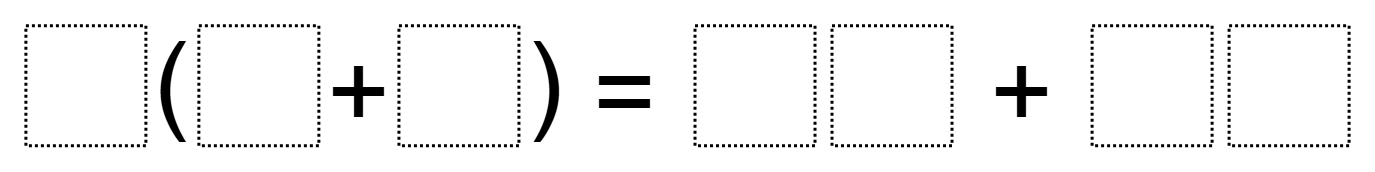Home > Grade 6 > Expressions & Equations > Distributive Property 2

# Distributive Property 2

Directions: Fill in the boxes below using the whole numbers 0 through 9 no more than one time each so that you can make a true equation.### Hint

What number would be good/bad to distribute?

There are many possible answers. Here are some possible answers:
4(5 + 9) = 20 + 36
6 (4 + 5) = 24 + 30
8 (2 + 5) = 16 + 40
7 (3 + 8) = 21 + 56
9 (2 + 4) = 18 + 36

## Subtracting Multi-Decimals

Directions: Use the digits 1 to 9, at most one time each, to fill in …

1.My students absolutely LOVED doing this. We had an awesome follow up question: what is the lowest value for each expression you can get? What is the highest? Our lowest was 20. Our highest, 108. We had a lot of fun. Thanks for the challenge!

•Ted, when you say “lowest value for each expression,” are you talking about the expression on either side of the equation? Because I’m puzzled how you could get 20, unless you allow a 2-digit number to start with 0. Otherwise, on the right, you’ll have a minimum of 1 ten plus 2 tens, or at least 30.

I like the idea of high/low, though! My lowest (for either side) is 45 and my highest is 126.

•Robert Kaplinsky

Thanks Ted and Julie. Ted, your version of the problem makes it a DOK 3 problem and you should definitely submit it to Open Middle using the link in the menu bar. That being said, definitely consider Julie’s feedback as it can be challenging writing the problem in such a way that it makes sense to virtually everyone.